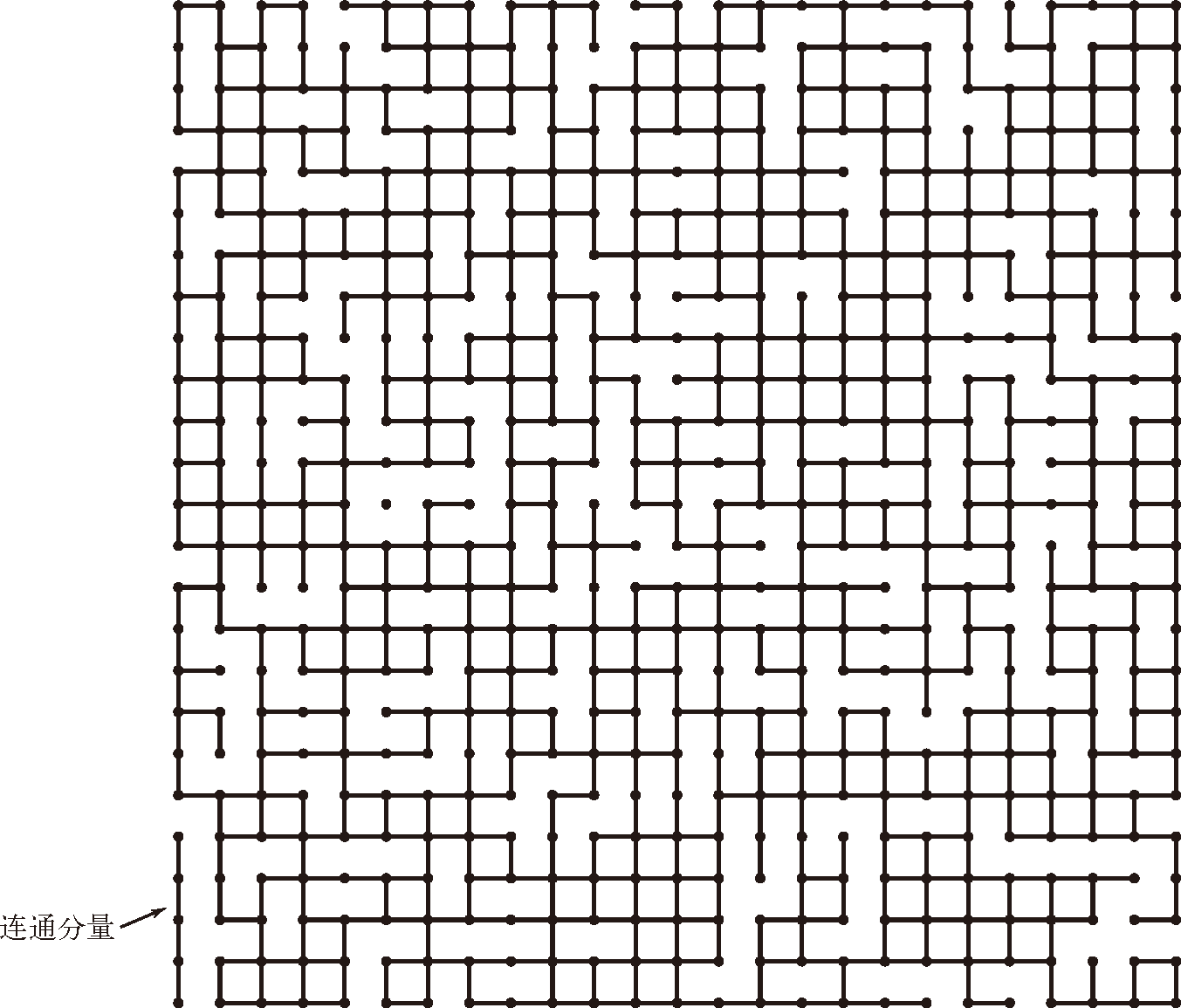# 算法（4th ed）(202)：基础——案例研究：union-find 算法 7.1.3(动态连通性：数学集合）public class UF              UF(int N) 以整数标识（0 到 $N-1$）初始化 $N$ 个触点       void   union(int p, int q) 在 p 和 q 之间添加一条连接        int   find(int p) p（0 到 $N-1$）所在的分量的标识符    boolean   connected(int p, int q) 如果 p 和 q 存在于同一个分量中则返回 true        int   count() 连通分量的数量

• 定义一种数据结构表示已知的连接；
• 基于此数据结构实现高效的 union()find()connected()count() 方法。

public class UF{  private int[] id;     // 分量 id（以触点作为索引）  private int count;    // 分量数量  public UF(int N)  {  // 初始化分量 id 数组     count = N;     id = new int[N];     for (int i = 0; i < N; i++)        id[i] = i;  }  public int count()  {  return count;  }  public boolean connected(int p, int q)  {  return find(p) == find(q);  }  public int  find(int p)  public void union(int p, int q)  // 请见 1.5.2.1 节用例（quick-find）、1.5.2.3 节用例（quick-union）和算法 1.5（加权 quick-union）  public static void main(String[] args)  {  // 解决由 StdIn 得到的动态连通性问题     int N = StdIn.readInt();              // 读取触点数量     UF uf = new UF(N);                    // 初始化 N 个分量     while (!StdIn.isEmpty())     {        int p = StdIn.readInt();        int q = StdIn.readInt();           // 读取整数对        if (uf.connected(p, q)) continue;  // 如果已经连通则忽略        uf.union(p, q);                    // 归并分量        StdOut.println(p + " " + q);      // 打印连接     }     StdOut.println(uf.count() + "components");  }}

% java UF < tinyUF.txt4 33 86 59 42 15 07 26 12 components

% more tinyUF.txt104 33 86 59 42 18 95 07 26 11 06 7 % more mediumUF.txt625528 503548 523...900 条连接 % more largeUF.txt1000000786321 134521696834 98245...200 万条连接

union-find 的成本模型。在研究实现 union-find 的 API 的各种算法时，我们统计的是数组的访问次数（访问任意数组元素的次数，无论读写）。